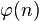# Number Theory - Euler's Phi Function

Euler's phi function is an important property in Number Theory. But before we go in any detail into this topic, let me clarify that it is very unlikely that a CAT question will require students to know Euler's Phi function. Before, we go into Euler's Phi function, it is probably good to have a look at the mod function. There is a simple blog post here.
As ever, wikipedia gives an excellent starting point here .$\varphi(n)$ or phi(n) is defined as the number of natural numbers less than or equal to n and are coprime to n.
phi(4) = 2 (The numbers 1 and 3)
phi(12) = 4 (The numbers 1, 5, 7 and 11)
phi(13) = 12 (All numbers below 13)
In general phi(p) = p-1 when is a prime.
Generalising further, when N = paqbrc
phi(N) = N (1-1/p) * (1-1/q) * (1-1/r) (The proof for this is intuitive enough. Keep eliminating all numbers that are not coprime from 1 to N-1)
Now, Euler's phi function states this
For two numbers m,n that are coprime (HCF of m,n =1)
m phi(n) = 1 mod n, (m phi(n) leaves a remainder of 1 when divided by n)

m phi(n) – 1 is a multiple of n
When we apply it in a scenario where n is prime, we get

m p-1 = 1 mod p
m p-1 – 1 is a multiple of n
This last observation is also called Fermat's Little Theorem . In many ways, Euler's phi function is an extension of Fermat's Little Theorem.
Excellent theorem, fairly clear implications whenever a question on remainders is asked. For instance if a question states What is the remainder when 100^56 is divided by 29, we can straight away see that the answer is 1. Having said that, I do not think that a CAT question will require students to know Euler's phi function and properties. Any question that gets simplified using Euler's phi function will have a simpler alternate solution as well. Even if you solve using Euler's phi function, kindly look for the alternate method.
The proof for Euler's Phi function and/or Fermat's Little Theorem can be found here

The proof for Wilson's Theorem can be seen here
IIM CAT Preparation Tips: Number Theory - Euler's Phi Function

## Nov 15, 2010

### Number Theory - Euler's Phi Function

Euler's phi function is an important property in Number Theory. But before we go in any detail into this topic, let me clarify that it is very unlikely that a CAT question will require students to know Euler's Phi function. Before, we go into Euler's Phi function, it is probably good to have a look at the mod function. There is a simple blog post here.
As ever, wikipedia gives an excellent starting point here .$\varphi(n)$ or phi(n) is defined as the number of natural numbers less than or equal to n and are coprime to n.
phi(4) = 2 (The numbers 1 and 3)
phi(12) = 4 (The numbers 1, 5, 7 and 11)
phi(13) = 12 (All numbers below 13)
In general phi(p) = p-1 when is a prime.
Generalising further, when N = paqbrc
phi(N) = N (1-1/p) * (1-1/q) * (1-1/r) (The proof for this is intuitive enough. Keep eliminating all numbers that are not coprime from 1 to N-1)
Now, Euler's phi function states this
For two numbers m,n that are coprime (HCF of m,n =1)
m phi(n) = 1 mod n, (m phi(n) leaves a remainder of 1 when divided by n)

m phi(n) – 1 is a multiple of n
When we apply it in a scenario where n is prime, we get

m p-1 = 1 mod p
m p-1 – 1 is a multiple of n
This last observation is also called Fermat's Little Theorem . In many ways, Euler's phi function is an extension of Fermat's Little Theorem.
Excellent theorem, fairly clear implications whenever a question on remainders is asked. For instance if a question states What is the remainder when 100^56 is divided by 29, we can straight away see that the answer is 1. Having said that, I do not think that a CAT question will require students to know Euler's phi function and properties. Any question that gets simplified using Euler's phi function will have a simpler alternate solution as well. Even if you solve using Euler's phi function, kindly look for the alternate method.
The proof for Euler's Phi function and/or Fermat's Little Theorem can be found here

The proof for Wilson's Theorem can be seen here